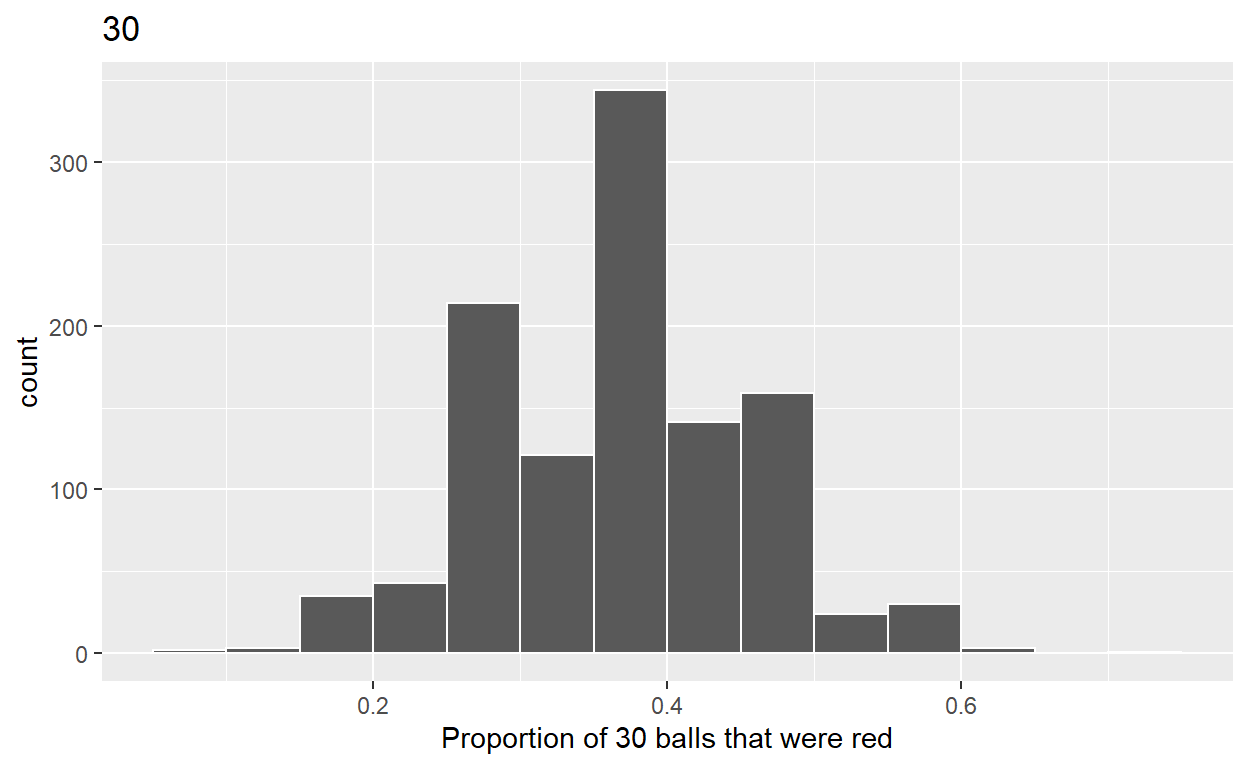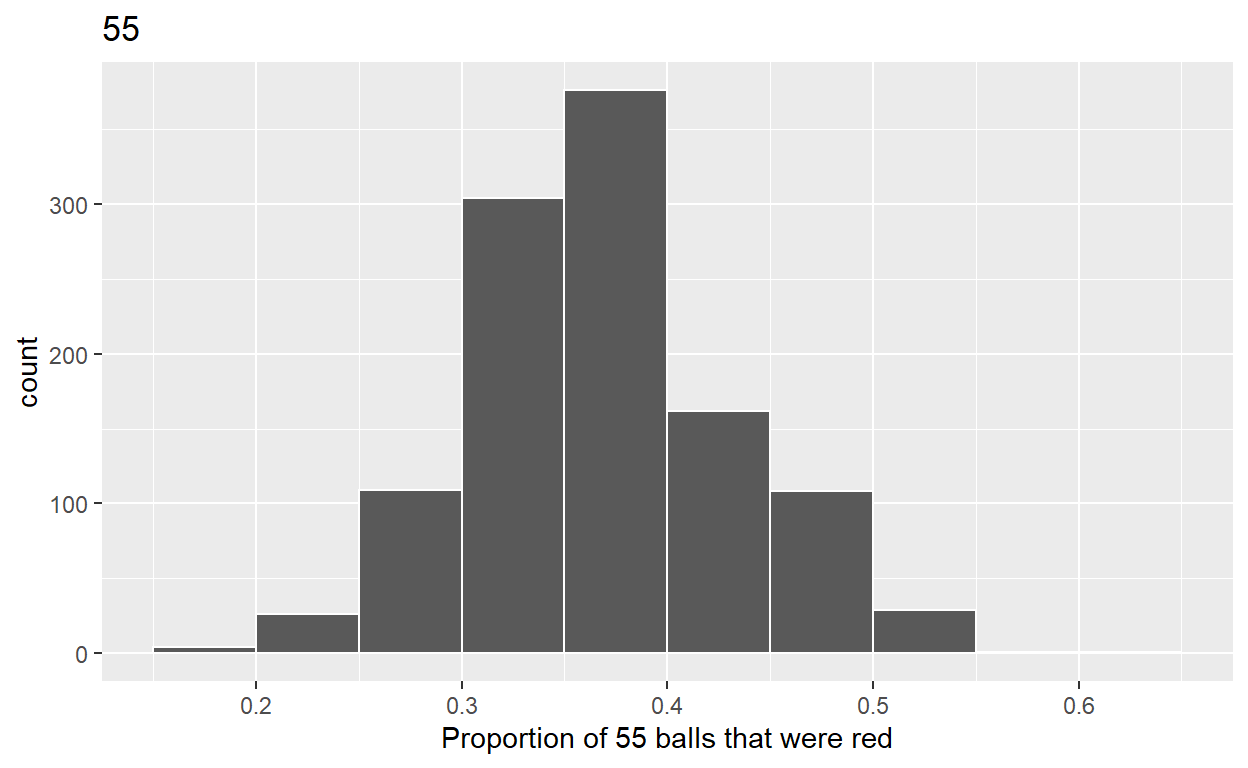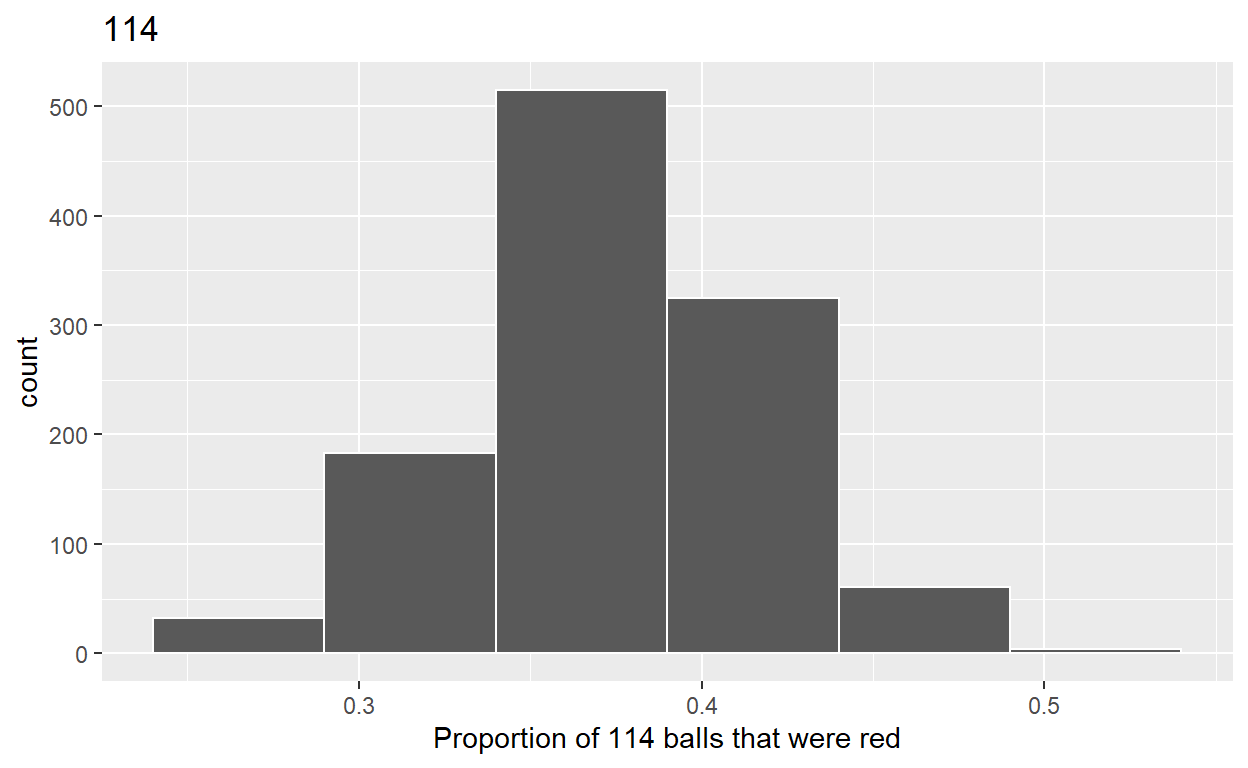# Sampling

Based on Chapter 7 of ModernDrive. Code for Quiz 11.

1. Load the R package we will use.
``````library(tidyverse)
``````
1. Quiz questions
• Replace all the instances of ‘SEE QUIZ’. These are inputs from your moodle quiz.

• Replace all the instances of ‘???’. These are answers on your moodle quiz.

• Run all the individual code chunks to make sure the answers in this file correspond with your quiz answers

• After you check all your code chunks run then you can knit it. It won’t knit until the ??? are replaced

• The quiz assumes that you have watched the videos and worked through the examples in Chapter 7 of ModernDive

# Question:

7.2.4 in Modern Dive with different sample sizes and repetitions

• Make sure you have installed and loaded the tidyverse and the moderndive packages

• Fill in the blanks

• Put the command you use in the Rchunks in your Rmd file for this quiz.

Modify the code for comparing different sample sizes from the virtual bowl

Segment 1: sample size = 30

1.a) Take 1120 samples of size of 30 instead of 1000 replicates of size 25 from the bowl dataset. Assign the output to virtual_samples_30

``````virtual_samples_30 <- bowl %>%
rep_sample_n(size = 30, reps = 1120)
``````

1.b) Compute resulting 1120 replicates of proportion red

• group_by replicate THEN

• create variable red equal to the sum of all the red balls

• create variable prop_red equal to variable red / 30

• Assign the output to virtual_prop_red_30

``````virtual_prop_red_30 <- virtual_samples_30 %>%
group_by(replicate) %>%
summarize(red = sum(color == "red")) %>%
mutate(prop_red = red / 30)
``````

1.c) Plot distribution of virtual_prop_red_30 via a histogram use labs to

• label x axis = “Proportion of 30 ball that were red”

• create title = “30”

``````ggplot(virtual_prop_red_30, aes(x = prop_red)) +
geom_histogram(binwidth = 0.05, boundary = 0.4, color = "white") +
labs(x = "Proportion of 30 balls that were red", title = "30")
``````Segment 2: sample size = 55

2.a) Take 1120 samples of size of 55 instead of 1000 replicates of size 50. Assign the output to virtual_samples_55

``````virtual_samples_55 <- bowl %>%
rep_sample_n(size = 55, reps = 1120)
``````

2.b) Compute resulting 1120 replicates of proportion red

• group_by replicate THEN

• create variable red equal to the sum of all the red balls

• create variable prop_red equal to variable red / 55

• Assign the output to virtual_prop_red_55

``````virtual_prop_red_55 <- virtual_samples_55 %>%
group_by(replicate) %>%
summarize(red = sum(color == "red")) %>%
mutate(prop_red = red / 55)
``````

2.c) Plot distribution of virtual_prop_red_55 via a histogram use labs to

• label x axis = “Proportion of 55 balls that were red”

• create title = “55”

``````ggplot(virtual_prop_red_55, aes(x = prop_red)) +
geom_histogram(binwidth = 0.05, boundary = 0.4, color = "white") +
labs(x = "Proportion of 55 balls that were red", title = "55")
``````Segment 3: sample size = 114

3.a) Take 1120 samples of size of 114 instead of 1000 replicates of size 50. Assign the output to virtual_samples_114

``````virtual_samples_114 <- bowl %>%
rep_sample_n(size = 114, reps = 1120)
``````

3.b) Compute resulting 1120 replicates of proportion red

• group_by replicate THEN

• create variable red equal to the sum of all the red balls

• create variable prop_red equal to variable red / 114

• Assign the output to virtual_prop_red_114

``````virtual_prop_red_114 <- virtual_samples_114 %>%
group_by(replicate) %>%
summarize(red = sum(color == "red")) %>%
mutate(prop_red = red / 114)
``````

3.c) Plot distribution of virtual_prop_red_114 via a histogram use labs to

• label x axis = “Proportion of 114 balls that were red”

• create title = “114”

``````ggplot(virtual_prop_red_114, aes(x = prop_red)) +
geom_histogram(binwidth = 0.05, boundary = 0.04, color = "white") +
labs(x = "Proportion of 114 balls that were red", title = "114")
``````Calculate the standard deviations for your three sets of 1120 values of prop_red using the standard deviation

n = 30

``````virtual_prop_red_30 %>%
summarize(sd = sd(prop_red))
``````
``````# A tibble: 1 x 1
sd
<dbl>
1 0.0895``````

n = 55

``````virtual_prop_red_55 %>%
summarize(sd = sd(prop_red))
``````
``````# A tibble: 1 x 1
sd
<dbl>
1 0.0639``````

n = 114

``````virtual_prop_red_114 %>%
summarize(sd = sd(prop_red))
``````
``````# A tibble: 1 x 1
sd
<dbl>
1 0.0432``````

The distribution with sample size, n = 114, has the smallest standard deviation (spread) around the estimated proportion of red balls.

``````ggsave(filename = "preview.png",
path = here::here("_posts", "2022-04-19-sampling"))
``````